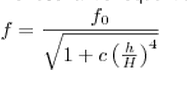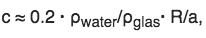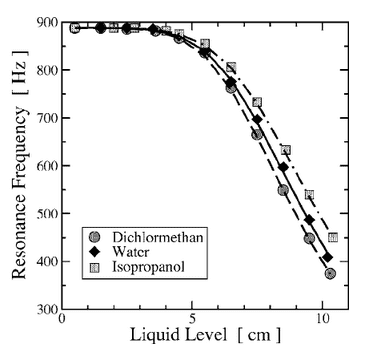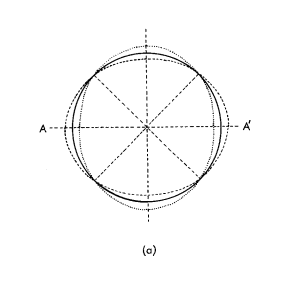# Resonating wineglass problem

I have done an experiment where I vary the levels of water in a wineglass and record the frequencies it produces which differ depending on the height of the water.

Below you see 1 equation, apparently I can use this equation to mathematically prove my results from my experiment, (this equation has been rewritten from the original equation created by AP french (https://www.nikhef.nl/~h73/tgo/praktgeluid/French1983.pdf)

My first question is: How has this equation been rewritten (where does the 0.2 come from etc. ) and what does the overall equation mean.
My second question is whether the variables that I think they are are actually the variables that they are.
I think the variables are: (could someone please confirm)
h = height of water in the wineglass
H= height of the wineglass
a= thickness of glass
f0= frequency of wineglass when emptyR = the radius of the glass
my problem is that the radius of the glass differs it is bigger in the middle etc. so which radius do I use???
for now with the calculations I've done I've just used the radius of the glass at the top of the glass.

Another question is graph related my graph currently looks something like this:

I've also read that its possible to plot two parts of the equation above against each other which would create a linear relationship in a graph and this would then prove the equation again. (could someone explain how to do this and why this works?) (this is also explained in ap french document i have posted url to earlier but i don't understand what is written there.)(ignore the stuff in the middle this is not my graph)

Finally I understand that the reason for the frequency to decrease has to do with nodes and antinodes and standing waves. basically the glass vibrates and the water within the glass vibrates with it. And this has to do with kinetic energy etc.#### Attachments

Last edited by a moderator:

scottdave
Homework Helper
In the paper, a diagram of a glass with straight sides is shown, so I understand your confusion about the diameter of your curved glass. But the paper also states that most of the deforming (from circle to ellipse) seems to happen at the rim. This would support using the measured dimension at the rim. I am not sure about the 0.2 factor. It may have something to do with the shape of the wineglass?

haruspex
Homework Helper
Gold Member
2020 Award
The 0.2 (i.e. 1/5) can be traced back to the 5/8 in equation 5. It arose there as the result of an integration.

haruspex
Homework Helper
Gold Member
2020 Award
most of the deforming (from circle to ellipse) seems to happen at the rim.
Yes, but if the top of the liquid is at a significantly wider part then this will affect the calculation of its KE.

Yes, but if the top of the liquid is at a significantly wider part then this will affect the calculation of its KE.
Could you explain the relationship (frequency decreases as water level rises) in relation to kinetic and potential energy.

haruspex
Homework Helper
Gold Member
2020 Award
Could you explain the relationship (frequency decreases as water level rises) in relation to kinetic and potential energy.
In SHM, potential energy is proportional to the square of the displacement, while KE is proportional to the square of the speed. The sum, ##A\dot x^2+Bx^2##, is constant. Writing ##x=c \sin(\omega t)##, we find ##B\sin^2(\omega t)+A\omega^2\cos^2(\omega t)## is constant, so ##\omega^2=\frac BA##.
Adding liquid increases the KE factor, A, so decreases the frequency.

In the same way, lengthening a pendulum increases the inertial factor.

In SHM, potential energy is proportional to the square of the displacement, while KE is proportional to the square of the speed. The sum, ##A\dot x^2+Bx^2##, is constant. Writing ##x=c \sin(\omega t)##, we find ##B\sin^2(\omega t)+A\omega^2\cos^2(\omega t)## is constant, so ##\omega^2=\frac BA##.
Adding liquid increases the KE factor, A, so decreases the frequency.

In the same way, lengthening a pendulum increases the inertial factor.

Thank you this is quite a mathematical explanation could you also explain it scientifically?

haruspex
Homework Helper
Gold Member
2020 Award
Thank you this is quite a mathematical explanation could you also explain it scientifically?
Maths is not scientific?
I can give a more intuitive explanation. The PE involved is related to the distance moved, and hence to the amplitude, independently of the mass. But the KE depends on both the mass and the speed. Since these are the same, a greater mass for the same amplitude means a lower speed, so longer period.

Maths is not scientific?
I can give a more intuitive explanation. The PE involved is related to the distance moved, and hence to the amplitude, independently of the mass. But the KE depends on both the mass and the speed. Since these are the same, a greater mass for the same amplitude means a lower speed, so longer period.

Yes intuitive was what I meant of course, thank you it makes more sense now.

After understanding this concept a little better another question has arisen:

The frequency of the sound you hear coming from a wineglass when you hit it with a pen for example is its natural frequency. Now from what I understand if you were to continuously emit a force such as from a loudspeaker at this frequency near the wineglass it would eventually shatter as it would vibrate back and forth at its maximum amplitude and reach its maximum elastic capacity. But then when you fill the wine glass with a little bit of water the frequency changes: it goes down, does this also mean that to be able to break the glass now you would have to emit a sound that is the same as its resonance frequency at that specific water level. For the glass to shatter?

haruspex
Homework Helper
Gold Member
2020 Award
you would have to emit a sound that is the same as its resonance frequency at that specific water level. For the glass to shatter
Yes. Of course, the oscillation is damped, so the sound source has to have enough power to overcome the damping.

rude man
Homework Helper
Gold Member
This is off-subject but you might be interested in another aspect of a vibrating wineglass: if you excite the rim at say 0 degrees with a hammer, you get nodes and antinodes at that angle. But turn the glass 90 degrees and you will notice that the nodes follow only part-way, about 60 degrees. The remaining 30 degrees makes the glass a sort of gyroscope, remembering which way is 'north' as you turn 'east' for example. In fact, such a gyro has been produced and is colloquially and fittingly enough named the "wineglass gyro".

Yes. Of course, the oscillation is damped, so the sound source has to have enough power to overcome the damping.

Does this also mean that a wineglass that is partially filled will have different harmonics from an empty wineglass. ?
As an empty wineglass won't only shatter at its natural frequency but also multiple harmonics of this frequency right?

haruspex
Homework Helper
Gold Member
2020 Award
Does this also mean that a wineglass that is partially filled will have different harmonics from an empty wineglass. ?
Do you mean, would the harmonics bear the same relationship to the fundamental in a partly filled glass as in an empty one? Largely, I would think, but the liquid might skew the power distribution towards the lower frequencies.
As an empty wineglass won't only shatter at its natural frequency but also multiple harmonics of this frequency right?
Yes, but typically it would take more power at the higher frequencies I believe.

Also, the harmonics might not be what you expect. What would the fundamental mode be? I can imagine the entire rim expanding and contracting in unison, the restoring force in that case being the elasticity of the glass. Another mode would be opposite sides move out while points 90 degrees around move in, the restoring force being flexural modulus. The frequency of this would be unrelated to the elasticity mode. A third would have antinodes 120 degrees apart moving in synchrony, maybe 3/2 times the frequency of the second, etc.

I assume the article you linked makes an assumption about the fundamental mode, but I did not read that part. I think it had the liquid surface rippling in concentric rings, suggesting the elastic mode for the glass.

Nidum
Gold Member

+ and other videos which follow on .

Do you mean, would the harmonics bear the same relationship to the fundamental in a partly filled glass as in an empty one? Largely, I would think, but the liquid might skew the power distribution towards the lower frequencies.

Yes, but typically it would take more power at the higher frequencies I believe.

Also, the harmonics might not be what you expect. What would the fundamental mode be? I can imagine the entire rim expanding and contracting in unison, the restoring force in that case being the elasticity of the glass. Another mode would be opposite sides move out while points 90 degrees around move in, the restoring force being flexural modulus. The frequency of this would be unrelated to the elasticity mode. A third would have antinodes 120 degrees apart moving in synchrony, maybe 3/2 times the frequency of the second, etc.

I assume the article you linked makes an assumption about the fundamental mode, but I did not read that part. I think it had the liquid surface rippling in concentric rings, suggesting the elastic mode for the glass.

Yes that's roughly what I meant.

Now I understand you get a standing wave pattern on the surface of the water (and the rim of the glass moving back and forth) , when you apply force to a wineglass by moving your finger across the rim (you are constantly applying a force)
Or with a loudspeaker you are constantly applying a force which creates nodes and antinodes (where waves intersect and cancel is at the nodes and where waves add up is at the antinodes)

When you apply a force by hitting the wineglass once ( there is only one wave ..... so this would not create a standing wave pattern right? ) but what is causing it to emit a sound.

haruspex
Homework Helper
Gold Member
2020 Award
when you apply force to a wineglass by moving your finger across the rim (you are constantly applying a force)
It's not a constant force. It keeps flipping between static friction and the weaker kinetic friction. A bow on a violin string works the same way. When static friction, the bow pulls the string sideways. The changing angle of the tension means that the component perpendicular to the string swiftly overcomes friction and the string slides back to almost straight, whereupon static friction takes over again.
The constant oscillation of the force provides input power.
When you apply a force by hitting the wineglass once ( there is only one wave ..... so this would not create a standing wave pattern right? ) but what is causing it to emit a sound.
The impulse sets it vibrating, just as for a tuning fork, a bell, a drum... The vibration will die away gradually.

It's not a constant force. It keeps flipping between static friction and the weaker kinetic friction. A bow on a violin string works the same way. When static friction, the bow pulls the string sideways. The changing angle of the tension means that the component perpendicular to the string swiftly overcomes friction and the string slides back to almost straight, whereupon static friction takes over again.
The constant oscillation of the force provides input power.

The impulse sets it vibrating, just as for a tuning fork, a bell, a drum... The vibration will die away gradually.

Ok so there is a standing wave formed due to the vibration...

Coming back to the energy concept to explain the decrease in frequency,

Due to the law of conservation of energy, the potential energy and kinetic energy must always add up to the same total amount of energy (the total energy remains constant)

so PE + KE = E total (which is constant no matter the water level of the wineglass)

The potential energy in this case is the elastic potential energy from the glass. (this remains the same also)

The kinetic energy is due to the vibration of the glass, there is both kinetic energy in the glass itself and kinetic energy from the liquid.

when we add more liquid the kinetic energy increases and you mentioned before that this means that the frequency must decrease as they are related (for the total energy to remain the same I'm assuming.)

If what I'm describing here is correct could you help me apply the equations you mentioned earlier to this concept again because the amount of variables and what they correspond to are a bit overwhelming to me.

haruspex
Homework Helper
Gold Member
2020 Award
when we add more liquid the kinetic energy increases and you mentioned before that this means that the frequency must decrease as they are related (for the constant energy to remain the same I'm assuming.)
Not quite. I could have done a better job of explaining that.
In SHM, energy shifts back and forth between KE and PE. Yes, the sum is constant, but the relevant fact here is that the variation of each must be the same, i.e. ΔE = max PE - min PE = max KE - min KE. If we fix the spring constant and ΔE then the distance amplitude is fixed. If at the same time we increase the inertia then the velocity amplitude must decrease. So distance/velocity increases and the period increases.

Not quite. I could have done a better job of explaining that.
In SHM, energy shifts back and forth between KE and PE. Yes, the sum is constant, but the relevant fact here is that the variation of each must be the same, i.e. ΔE = max PE - min PE = max KE - min KE. If we fix the spring constant and ΔE then the distance amplitude is fixed. If at the same time we increase the inertia then the velocity amplitude must decrease. So distance/velocity increases and the period increases.

I understand up to "min KE."

what does inertia mean?

And could you please specify which parts of the equation you're referring.

haruspex
Homework Helper
Gold Member
2020 Award
I understand up to "min KE."

what does inertia mean?

And could you please specify which parts of the equation you're referring.
In the wineglass context, inertia refers to the mass (glass and liquid). More liquid means more mass, so for a given KE max, less speed.

It will be easier to describe in a simpler model, a mass on a smooth horizontal surface, attached to a spring.
If the max displacement is D then the energy flowing back and forth between the two modes is ½kD2. If the mass (inertia) is m then that means the max speed is given by ½kD2 = ½mvmax2. If we keep k and D fixed but increase m then this means vmax must decrease. The period will be related to D/vmax (though not exactly that, of course), so will increase.

Ok the concept is starting to make more sense to me, if it wont bother you too much do you mind explaining this whole energy concept in the wineglass context one last time using super simple language and explaining what each variable in the equation is.

why the frequency decreases as water is added.

haruspex